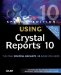# Understanding and Implementing Formulas

### Introduction

Chapter 2, "Selecting and Grouping Data," introduced the concept of formulas, and you saw how to create them and subsequently drop them into a report from the Field Explorer. This chapter explores the Formula Editor in more detail.

Formulas provide great flexibility and power when creating Crystal Reports by enabling you to create derived fields not directly stored in available data sources. Formulas also enable you to create advanced conditional object formatting and use flexible selection formulas in a report.

Crystal Reports has a number of built-in tools that facilitate the formula creation and formula reuse processes, the Formula Editor being a good example. The Formula Workshop provides a single convenient access point to almost all your formula fields within a given report. SQL Expression fields, Record and Group Selection formulas, Formatting formulas, and Custom Report- and Repository-based functions can all be accessed from the new Formula Workshop.

This chapter covers the following topics:

• An introduction to the Formula Workshop
• A review of the Formula Workshop Tree Elements
• Formula Editor
• Arithmetic, Date, and String formulas
• Type conversion
• Variables in formulas
• Formula Expert
• Formula Extractor
• Multi-pass ReportingSpecial Edition Using Crystal Reports 10
ISBN: 0789731134
EAN: 2147483647
Year: 2003
Pages: 341